# Arithmetic Series - Sum of n terms

A sequence is the set of the outputs of a function defined from the set of natural numbers to the set of real numbers or complex numbers. If the codomain of the function is the set of real numbers, it is called a real sequence and if it is the set of complex numbers, on the other hand, it is called a complex sequence. For example 1, 4, 9, 16, 25, 36, 49 ……….625 represents a sequence of squares of natural numbers till 25.

In very simple terms, a sequence is an ordered set of numbers. A sequence is denoted using braces. The following sequence represents all the natural numbers. A sequence can be finite or infinite depending upon the number of terms it can have.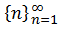Now that we know what a sequence is let us learn about series. Sequence and series are very often confused with each other. Series are derived from sequences. In this article, we are going to discuss the sum of n terms of an arithmetic series with formulas and examples.

## What is Arithmetic Series?

A series is defined as the sum of the terms of a sequence. It is denoted by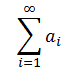Where ai is the ith term of the sequence and I is a variable. ∑ is a symbol which stands for ‘summation’. It was invented by Leonard Euler, a Swiss mathematician.

The meaning of the above expression written using summation is: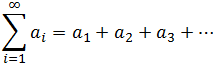## Sum of N terms of an Arithmetic Series

Let us now discuss some special arithmetic series and their sum.

### Case 1: Sum of “n” Natural Numbers

• 1 + 2 + 3 + 4 + ………. + n

This arithmetic series represents the sum of n natural numbers. Let us try to calculate the sum of this arithmetic series.

The difference between the sum of n natural numbers and sum of (n – 1) natural numbers is n, i.e.

Sn – Sn-1 = n

Let us now proceed by taking the difference of sum of n natural numbers and sum of (n -2) natural numbers and so on,

Sn – Sn-2 = n + (n – 1) = 2n -1

Sn – Sn-3 = n + (n – 1) + (n – 2) = 3n – (1 + 2)

Sn – Sn-4 = n + (n – 1) + (n – 2) + (n – 3) = 4n – (1 + 2 + 3)

Proceeding in the same manner, the general term can be expressed as: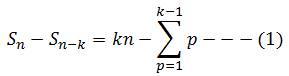According to the above equation the nth term is clearly kn and the remaining terms are sum of natural numbers preceding it.

Now if k = n, equation 1 can be written as: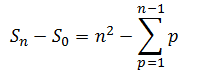Since S0 is 0 the above sum becomes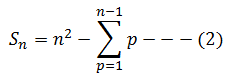Also, sigma (p to n-1) {p} is one n short of Sn

Therefore,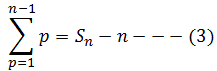Substituting the value of equation (3) in (2), we get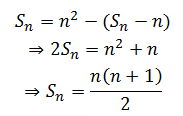### Case 2: Sum of Squares of “n” Natural Numbers

• 12 + 22 + 32 + 42 + ………. + n2

This arithmetic series represents the sum of squares of n natural numbers. Let us try to calculate the sum of this arithmetic series.

To prove this let us consider the identity p3 – (p – 1)3 = 3p2 – 3p + 1. In this identity let us put p = 1, 2, 3…. successively, we get

23 – (1 – 1)3 = 3(1)2 – 3(1) + 1

23 – (2 – 1)3 = 3(2)2 – 3(2) + 1

33 – (3 – 1)3 = 3(3)2 – 3(3) + 1

………………………………………..

………………………………………..

………………………………………..

n3 – (n – 1)3 = 3(n)2 – 3(n) + 1

Adding both the sides of the equation, we get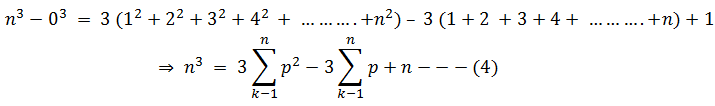We have already calculated the sum of n natural numbers as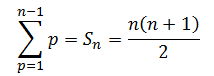Substituting this value n equation (4), we get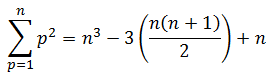This represents the sum of squares of natural numbers using the summation notation. It can be simplified as: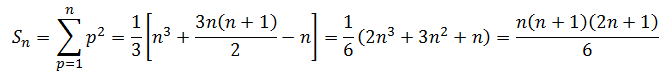### Case 3: Sum of cubes of “n” Natural Numbers

• 13 + 23 + 33 + 43 + ………. + n3

This arithmetic series represents the sum of cubes of n natural numbers. Let us try to calculate the sum of this arithmetic series.

To prove this let us consider the identity (p + 1)4 – p4 =4p3 + 6p2 + 4p + 1. In this identity let us put p = 1, 2, 3…. successively, we get

24 – 14 =4(1)3 + 6(1)2 + 4(1) + 1

34 – 24 =4(2)3 + 6(2)2 + 4(2) + 1

44 – 34 =4(3)3 + 6(3)2 + 4(3) + 1

……..………………………………………..

………..……………………………………..

…………..…………………………………..

(n + 1)4 – n4 =4n3 + 6n2 + 4n + 1

Adding both the sides of the equation, we get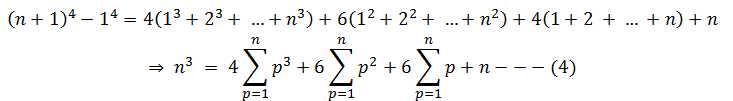We have already calculated the sum of n natural numbers and sum of squares of n natural numbers as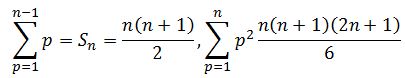Substituting these values in equation (4) and simplifying, we get;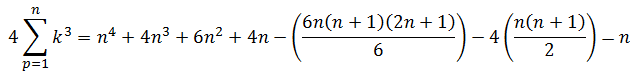This represents the sum of cubes of natural numbers using the summation notation. It can be simplified as: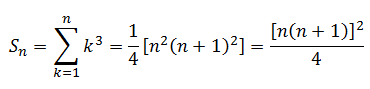Stay tuned with BYJU’S – The Learning App and download the app to learn all Maths-related concepts by exploring more videos.

## Frequently Asked Questions on Arithmetic Series – Sum of n-terms

Q1

### What is arithmetic series?

The arithmetic series is defined as the sum of all the terms of a given sequence.

Q2

### What is the sum of n terms of an arithmetic progression?

The formula to calculate the sum of n terms of AP is given as:
Sn= n/2[2a + (n – 1)d].
Where “a” is the first term, “d” is a common difference, and “n” is the number of terms.

Q3

### What is the formula for the sum of n natural numbers of an AP?

The formula to calculate the sum of “n” natural numbers of an AP is Sn = [n(n+1)]/2.

Q4

### What is the formula to calculate the sum of squares of n natural numbers?

The formula to calculate the sum of squares of “n” natural numbers is Sn= [n(n+1)(2n+1)]/6.

Q5

### What is the formula to calculate the sum of cubes of n natural numbers?

The formula to calculate the sum of cubes of “n” natural numbers is Sn = [n(n+1)/2]2.

Quiz on Arithmetic Series - Sum of n terms